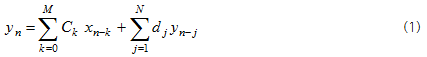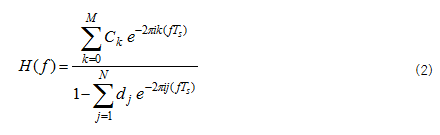IIR System means a system that, in addition to the present and past inputs, outputs generated in the previous step affect the present output values. This will be further explained using the following formula.Time series signals changing over time can be generated by signals precedent to near time. As you can see in the above formula (1), the signal to be generated, yn, is obtained by multiplying the precedent signal, xn-k , and the previously generated signal, yn-j, by constants, Ck and dj, and then summing them. In other words, the previously generated signal affects the next signal to be generated. Such signals generated are called as the recursive ones. Also, the signal system having such mechanism is called as recursive or Infinite Impulse System. And if the constant, d j, is zero (0) and so, the previously generated signal does not affect the next one to be generated, such signal is called as non-recursive one and such system is Finite Impulse System. In short, since IIR system can produce signals using some signals previously collected and signals immediately precedent to the signal to be generated, this system can simultaneously perform filtering as well as data collection, unlike FFT method requiring some specified block of data. So, real-time filtering is enabled in this system.
IIR (Infinite Impulse Response) FilterCorrespondence of the above formula (1) to the frequency band results in formula (2). In this formula (2), the type of filter can vary depending on constants, Ck and dj. Using this formula (2), constant is determined according to the shape of individual filter. For example, constants, Ck and dj, of Low Pass Filter are determined by calculating the formula (2) with a shape passing low frequency. If constants, Ck and dj, determined by this method are applied to the formula (1), IIR filter passing only low frequency is resulted.